### IMO Shortlist 2009 problem N5

Kvaliteta:
Avg: 0,0
Težina:
Avg: 8,0
Let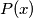$P\!\left(x\right)$ be a non-constant polynomial with integer coefficients. Prove that there is no function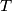$T$ from the set of integers into the set of integers such that the number of integers$x$ with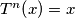$T^n\!\left(x\right) = x$ is equal to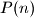$P\!\left(n\right)$ for every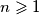$n \geqslant 1$, where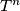$T^n$ denotes the$n$-fold application of$T$.

Proposed by Jozsef Pelikan, Hungary
Izvor: Međunarodna matematička olimpijada, shortlist 2009